# Introduction To Graphs Class 8 Notes- Chapter 15

According to the CBSE Syllabus 2023-24, this chapter has been renumbered as Chapter 13.

## What are the different types of Graphical Representation of Data?

Graphical methods used to present data are very efficient in organizing the data and understanding them. There are various graphical methods which are mentioned below:

• While comparing among categories, the method which is appropriate is the bar graph.
• While comparing parts of a whole, a pie chart is the ideal method.
• When data is provided in intervals, a histogram can be used for easier understanding.
• In the case of the data changing continuously over a period of time, a line graph will be useful
• When an unbroken line is represented, it is done with the help of a linear graph

To know more about Graphical Representation, visit here.

### Introduction to Graphs

Graphs are visual representation of data collected. Its purpose is to show numerical data in physical form so that it can be understood quickly, easily and clearly.

## Bar Graphs

### Bar Graph

A bar graph is used to show comparison among two or more different categories. Parallel vertical bars (rectangular in shape) are used to represent the data on a bar graph.

For example: The graph here represents a student’s marks in maths in the first, second and third terms respectively.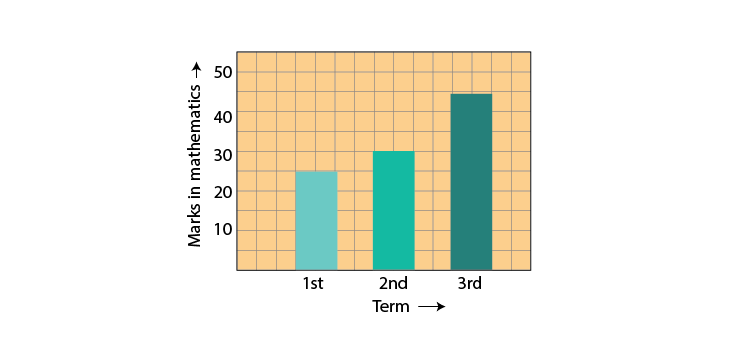#### For more information On Easiest way to Compare Quantities-Bar Graphs, Watch The Below Video:A bar graph can also have two or more bars to represent the same category like the example below.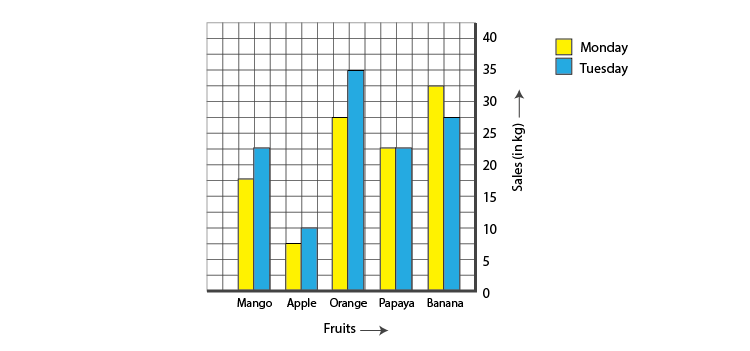#### For more information on Bar Graphs and Double Bar Graphs, watch the below videos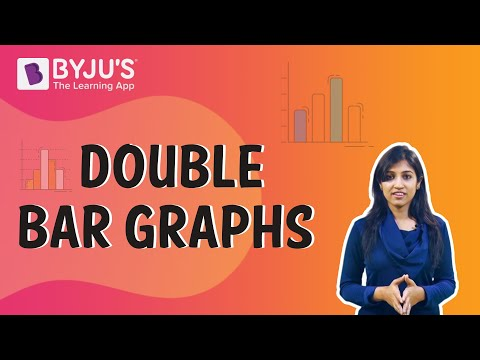To know more about Bar Graphs, visit here.

## Pie Charts

### Pie Charts

A pie graph is used to represent the parts of a whole. A circle is used to represent the whole.

The pie graph below is used to represent people’s choice of television channels. The circle as a whole here is represented by all the people who took part in the survey. Since it is a whole, the sum of all percentages represented in a pie graph must add up to 100%.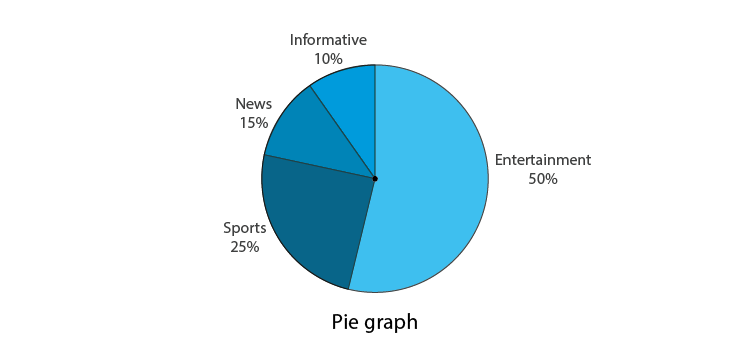To know more about Pie Chart, visit here.

## Histograms

### Histogram

A histogram is nothing but a bar graph, that is used to group numbers into ranges. It shows data in intervals like the case of the table below.

 Weight (kg) 40-45 45-50 50-55 55-60 60-65 No.of persons 4 12 13 6 5

The xaxis of the graph is labelled from 40-65, as Weights (in kg), in intervals of 5.  The yaxis is labelled as No. of persons.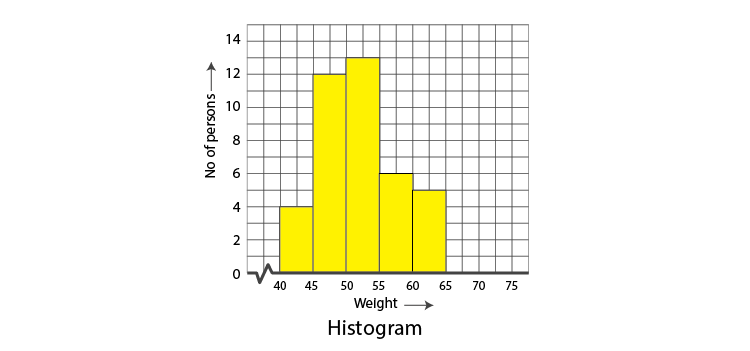A histogram is used to represent continuous data. In the graph above, it presents the data available for all values between 40 and 65.To know more about Histogram, visit here.

## Linear Graphs and Application

### Linear Graph

A graph where all the data points can be plotted on a single straight line is called a linear graph.

For any two variables, the relation can be drawn by constructing the table of values if the rule for that relation is mentioned. At least two points coordinates should be known to plot a straight line graph. These points must fit the rule.

For example, take the points W(2,6), X(3,5), Y(5,3) and Z(6,2). Upon plotting the points on the graph, we see that all of them can be connected by a straight line.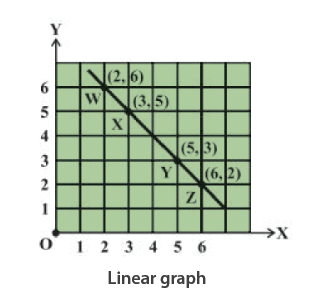To know more about Linear Graphs, visit here.

### Application of Graphs

Depending on how the values of a variable change with respect to another, we have two types : independent and dependent variable.

Independent (or control) variable is one where it’s value doesn’t change with respect to another quantity.

Dependent variable is one where the value does change with respect to another quantity.

For example, consider quantity of electricity consumed and the electricity bill. The quantity of electricity consumed doesn’t depend on any other quantity, hence it is an independent variable. The electricity bill however, can change with respect to the amount of electricity consumed, hence it is a dependent variable.

Graphs help to establish the relation between these two types of variables visually with the help of the cartesian plane.

To know more about Applications of Linear Graphs, visit here.

## Line Graphs and Linear Graphs

### Line Graph

A line graph is one that is used to plot data that changes over a period of time.

Consider a table of the kind as shown here :

 Time 6 AM 10 AM 2 PM 6 PM Temperature 37 40 38 35

Here as observed, the temperatures constantly varies over a period of time. So a line-graph can be used to chart the increase and decrease of temperature over the course of 12 hours from 6AM to 6PM. Time is on the x-axis and temperature will be on the y-axis.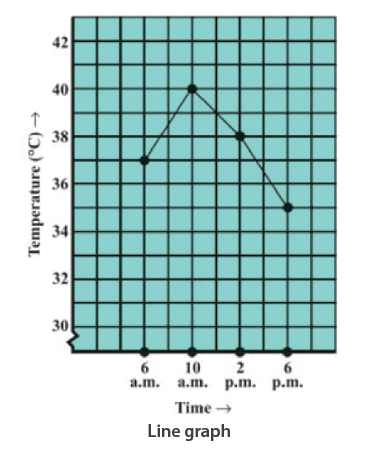To know more about Line Graph, visit here.

### Cartesian Plane and Coordinate Axes

A cartesian plane is formed and defined by two perpendicular number lines : the xaxis, which is horizontal  and the yaxis, which is vertical. These are called the coordinate axes.

The point at which the two axes meet is called the zero or origin of the cartesian plane.

The two coordinate axes help to plot any point on the cartesian plane.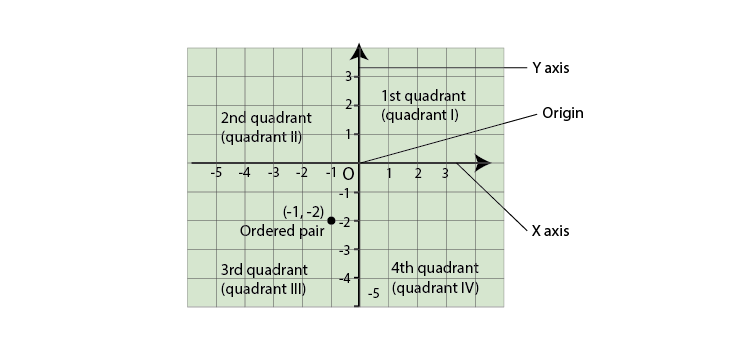To know more about Cartesian Plane, visit here.

### Representation of Point on the Plane

Ploting a point on the plane.

An ordered pair of numbers are used to represent any given point on a cartesian plane. They are written in the form (x,y), where the value of x represents the x-coordinate of the point and the value of y represents the y-coordinate of the point.

In simple terms, the x and y coordinates explain how far from the origin the point is with respect to the xaxis and yaxis respectively.
For example, consider a point (3,4). Here 3 is the x-coordinate while 4 is the y-coordinate. This means the point (3,4) lies 3 units from the origin on the xaxis and 4 units from the origin on the yaxis. The point is then plotted as shown below.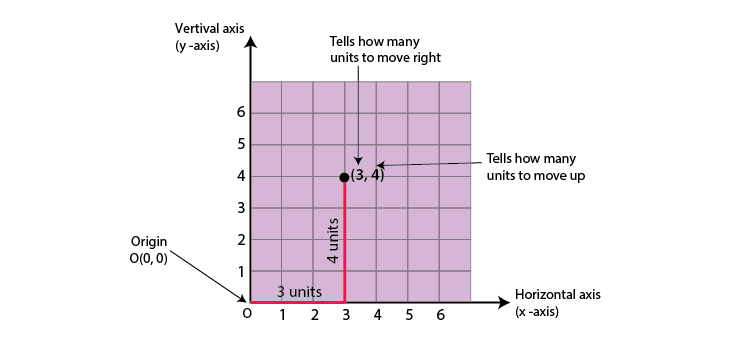To know more about Co-ordinates of a Point in 3D, visit here.

 Also Access NCERT Solutions for class 8 Maths Chapter 15 Application Of Linear Graphs

## Frequently asked Questions on CBSE Class 8 Maths Notes Chapter 15 Introduction to Graphs

Q1

### What are some of the uses of graphs?

1. Visualise data 2. Express complex data in simple format 3. Value addition to presentations

Q2

### What is a Histogram?

A histogram is a graphical representation that organizes a group of data points into user-specified ranges.

Q3

### What is a Cartesian plane?

A Cartesian plane is a graph with two axes, one is called the x-axis and the other one is the y-axis.